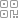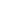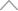6 3 0 4 2 6 0

# 【硬核干货】2500字全方面解读Python的格式化输出

Python

### % 占位符的使用

country_ = "France"

currency_ = "Euro"

print("%s is the currency of %s" % (currency_, country_))

output

Euro is the currency of France

%s

%f

%d

name = '欣一'

age = 24

height = 1.88

print('我是：%s, 年龄：%d, 身高：%fm' % (name,age,height))

output

2位小数

，代码如下

print('我是：%s, 年龄：%d, 身高：%.2fm' % (name,age,height))

output

## 左填充

display('%10s' % ('欣一'))

## 右填充

display('%-10s' % ('欣一'))

output

'        欣一'

'欣一        '

### f-string 格式化

country_ = "France"

currency_ = "Euro"

print(f"{currency_} is the currency of {country_}")

output

Euro is the currency of France

f-string

print(f'计算结果是：{2*10 + 3*15}')

output

65

Python

lambda

string_test = 'Python is awesome'

print(f'我想说 {string_test.lower()}')

output

a = 100

b = 10

print(f'计算的结果是：{(lambda x,y:x+y)(a,b)}')

output

format

### 不指定位置

print('我是：{}, 年龄：{}, 身高：{}m'.format (name,age,height))

output

### 指定位置

print('我是：{0}, 年龄：{1}, 身高：{2}m'.format (name,age,height))

print('我是：{0}, 年龄：{1}, 身高：{1}m'.format (name,age,height))

print('我是：{0}, 年龄：{2}, 身高：{1}m'.format (name,age,height))

output

### 关键字配对

print('我是：{name}, 年龄：{age}, 身高：{height}m'.format(name='欣一', age=25, height=1.88))

output

### 字典参数

dic = {'name':'欣一','age':24,'height':1.88}

print('我是：{name}, 年龄：{age}, 身高：{height}m'.format(**dic))

output

### 列表参数

foods = ['fish''beef''fruit']

s = 'i like eat {} and {} and {}'.format(*foods)

print(s)

output

i like eat fish and beef and fruit

foods = ['fish''beef''fruit']

s = 'i like eat {2} and {0} and {1}'.format(*foods)

print(s)

output

i like eat fruit and fish and beef

### 精度

pi = 3.1415926

print("{:.2f}".format(pi)) # 保留两位小数

print("{:+.3f}".format(pi)) # 带符号保留3位小数

print("{:.2%}".format(pi)) # 百分比保留两位小数

output

3.14

+3.142

314.16%

### 千分位分隔符

print('{:,}'.format(100000000))

output

100,000,000

print('\${:,}'.format(100000000))

output

\$100,000,000

## 新知精选

IT研发▪2021年度十佳创作者

### 优选课程 新知学院

•扫码下载商业新知APP~

•回到顶部